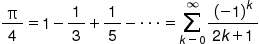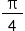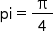## Overview

HUAWEI Accelerate Kit provides multi-thread programming APIs, enhancing the program performance and facilitating the development of multi-core and multi-thread applications.
The provided multi-thread programming APIs serve the following purposes:

• Creates and manages queues.
• Releases objects.

## What You Will Create

• In this codelab, you will implement multi-thread programming.

## What You Will Learn

• Perform multi-thread programming quickly using Accelerate Kit.
• Use Accelerate Kit APIs.
• Integrate the Accelerate SDK.
• Call the Accelerate Kit APIs.

## Hardware Requirements

• Windows, Linux, or Mac computer (desktop or laptop)
• Android mobile phone (with USB connection support)

## Software Requirements

• Java JDK 1.7 or later
• Android Studio 3.x
• Android (API level 24) or later

## Required Knowledge

• C language basics

To integrate HUAWEI Accelerate Kit, you must complete the following preparations:

1. Register as a developer.
2. Create an Android Studio project.
3. Generate a signing certificate fingerprint.
4. Configure the signing certificate fingerprint.

For details, please refer to "Integration Preparations" in Development Guide.

### 1. Create an Android Studio project.

Create a project named example in Android Studio, select Native C++, and use default settings in follow-up steps.### 2. Add the NDK build information.

Add the following lines for NDK build to the /app/build.gradle file in the project directory:

``````arguments "-DANDROID_STL=c++_shared"
abiFilters "arm64-v8a","armeabi-v7a"

``````### 3. Copy libraries and header files.

2. Copy the header files in the SDK to the resource library.
3. In the /app directory in the Android Studio project, create an include folder. Copy the files in the /include directory of the SDK to the newly created include folder.4. Copy the .so files in the SDK to the resource library.
5. Create a libs folder in the /app directory and create an arm64-v8a folder and an armeabi-v7a folder in the /app/libs directory.

Copy libdispatch.so and libBlockRuntime.so in th/lib64** 4 directory of the SDK to th/libs/arm64-v8a8a directory of Android Studio.

Copy the libdispatch.so and libBlockRuntime.so files in the /lib directory of the SDK to the /libs/armeabi-v7a directory of Android Studio.6. Modify the CMakeLists.txt file in the app/src/main/cpp directory as follows.
``````# For more information about using CMake with Android Studio, read the
# Sets the minimum version of CMake required to build the native library.
cmake_minimum_required(VERSION 3.4.1)
# Creates and names a library, sets it as either STATIC
# or SHARED, and provides the relative paths to its source code.
# You can define multiple libraries, and CMake builds them for you.
add_library( # Sets the name of the library.
native-lib
# Sets the library as a shared library.
SHARED
# Provides a relative path to your source file(s).
native-lib.cpp )
# Searches for a specified prebuilt library and stores the path as a
# variable. Because CMake includes system libraries in the search path by
# default, you only need to specify the name of the public NDK library
# you want to add. CMake verifies that the library exists before
# completing its build.
target_include_directories(
native-lib
PRIVATE
\${CMAKE_SOURCE_DIR}/../../../include)
find_library( # Sets the name of the path variable.
log-lib
# Specifies the name of the NDK library that
# you want CMake to locate.
log )
dispatch
SHARED
IMPORTED)
set_target_properties(
dispatch
PROPERTIES IMPORTED_LOCATION
\${CMAKE_SOURCE_DIR}/../../../libs/\${ANDROID_ABI}/libdispatch.so)
BlocksRuntime
SHARED
IMPORTED)
set_target_properties(
BlocksRuntime
PROPERTIES IMPORTED_LOCATION
\${CMAKE_SOURCE_DIR}/../../../libs/\${ANDROID_ABI}/libBlocksRuntime.so)
TARGET native-lib POST_BUILD
COMMAND
\${CMAKE_COMMAND} -E copy
\${CMAKE_SOURCE_DIR}/../../../libs/\${ANDROID_ABI}/libdispatch.so
\${CMAKE_LIBRARY_OUTPUT_DIRECTORY}/
COMMAND
\${CMAKE_COMMAND} -E copy
\${CMAKE_SOURCE_DIR}/../../../libs/\${ANDROID_ABI}/libBlocksRuntime.so
\${CMAKE_LIBRARY_OUTPUT_DIRECTORY}/
)
target_compile_options(native-lib PRIVATE -fblocks)
# can link multiple libraries, such as libraries you define in this
# build script, prebuilt third-party libraries, or system libraries.
target_link_libraries( # Specifies the target library.
native-lib
dispatch
BlocksRuntime
# Links the target library to the log library
# included in the NDK.
\${log-lib} )

``````

The preceding information is added and modified. Related parameters are described as follows:

• set_target_properties: specifies the path of the .so file related to the multi-thread library.
• target_compile_option: adds the -fblocks compilation option.

This section provides instructions for calculating π with the Accelerate Kit API. The Gregory-Leibniz infinite series is used to approximateπ as follows:To begin with, break the calculation down into eight tasks (task0–task7), so that a part of the infinite series is calculated each task, and the value ofcan be obtained by accumulating the result of each task. Then, the value of π is obvious.

1. Set related parameters, including the number of terms (n), total number of tasks (threads), and initialization of the calculation result (pi). Because pi needs to be updated and modified in a subsequent block, the modifier __block is added.
``````_block double pi = 0;
int n = 1000000;

``````
2. Call dispatch_queue_create to create a serial queue for π calculation. Then, call dispatch_apply to synchronously execute a task for multiple times in a specified queue. Generally, you are advised to set the specified queue to DISPATCH_APPLY_AUTO, which is a global concurrent queue whose priority is closest to that of the current queue. Since tasks are executed concurrently, and the π calculation in this example is reentrant, it is possible to accelerate the calculation in a manner of concurrent execution.
``````dispatch_queue_t accumulator = dispatch_queue_create("Compute pi", NULL);
// Step-by-step calculation of π
});

``````
3. Implement the calculation algorithm of π. That is, allocate the** n** terms into threads tasks (threads = 8). The start and end of each task are calculated based on the task ID (idx) of the current task, which is 0, 1, ..., or 7 in this example. flag indicates the plus or minus symbol of each term. sum indicates the accumulation result of the current task.
``````int start = idx * (n / threads);
int end = (idx + 1) * (n / threads);
double sum = 0;
for (int k = start; k < end; k++) {
double flag = (k & 1) ? -1.0 : 1.0;
sum += flag / (2 * k + 1);
}

``````
4. Call dispatch_sync to calculate the sum of each task. Then, obtain by accumulating the results of the eight tasks.``````dispatch_sync(accumulator, ^{
pi += sum;
});

``````
5. Call dispatch_release to release the space of the accumulator. Objects created by APIs of class dispatch_xxx_create() each correspond to a space on the heap. Because there is no garbage collection mechanism, such objects created by the user need to be proactively released when the user determines that the objects are no longer needed.
``````dispatch_release(accumulator);

``````
6. Output the accumulated string for π to the screen for display. π is kept to 20 decimal places.
``````extern "C" JNIEXPORT jstring JNICALL
Java_com_example_example_MainActivity_stringFromJNI(
JNIEnv* env,
jobject /* this */)
{
std::stringstream ss;
ss << std::setprecision(20) << calculate_pi();
return env->NewStringUTF(ss.str().c_str());
}

``````
7. Accelerated computation of π is complete. The following gives the complete code (app/src/main/cpp/native-lib.cpp).

``````#include <jni.h>
#include <string>
#include <iostream>
#include <sstream>
#include <iomanip>
#include <fcntl.h>
#include <android/log.h>
#include <dispatch/dispatch.h>

double calculate_pi(void)
{
__block double pi = 0;
int n = 1000000;
dispatch_queue_t accumulator = dispatch_queue_create("Compute pi", NULL);

int start = idx * (n / threads);
int end = (idx + 1) * (n / threads);
double sum = 0;
for (int k = start; k < end; k++) {
double flag = (k & 1) ? -1.0 : 1.0;
sum += flag / (2 * k + 1);
}

dispatch_sync(accumulator, ^{
pi += sum;
});
});

dispatch_release(accumulator);
return pi * 4;
}
extern "C" JNIEXPORT jstring JNICALL
Java_com_example_example_MainActivity_stringFromJNI(
JNIEnv* env,
jobject /* this */)
{
std::stringstream ss;
ss << std::setprecision(20) << calculate_pi();
return env->NewStringUTF(ss.str().c_str());
}

``````

Well done. You have successfully completed this codelab and learned how to:

• Complete multi-thread programming quickly with Accelerate Kit.
• Use Accelerate Kit APIs.
• Integrate the Accelerate SDK.
• Call the Accelerate Kit APIs.